Updating search results...

# 7 Results

View
Selected filters:
• UT.MATH.III.SIII.MP.7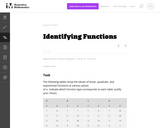Unrestricted Use
CC BY
Rating
0.0 stars

This task emphasizes the expectation that students know linear functions grow by constant differences over equal intervals and exponential functions grow by constant factors over equal intervals.

Subject:
Mathematics
Secondary Mathematics
Material Type:
Activity/Lab
Provider:
Illustrative Mathematics
Provider Set:
Illustrative Mathematics
Author:
Illustrative Mathematics
05/01/2012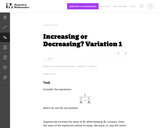Unrestricted Use
CC BY
Rating
0.0 stars

Students are asked to consider the expression that arises in physics as the combined resistance of two resistors in parallel. However, the context is not explicitly considered here. The task is good general preparation for problems more specifically aligned to either A-SSE.1 or A-SSE.2.

Subject:
Mathematics
Secondary Mathematics
Material Type:
Activity/Lab
Provider:
Illustrative Mathematics
Provider Set:
Illustrative Mathematics
Author:
Illustrative Mathematics
05/01/2012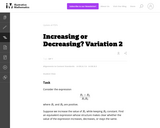Unrestricted Use
CC BY
Rating
0.0 stars

The purpose of this task is to help students see manipulation of expressions as an activity undertaken for a purpose.

Subject:
Mathematics
Secondary Mathematics
Material Type:
Activity/Lab
Provider:
Illustrative Mathematics
Provider Set:
Illustrative Mathematics
Author:
Illustrative Mathematics
05/01/2012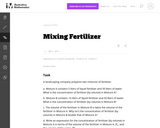Unrestricted Use
CC BY
Rating
0.0 stars

The problem deals with a rational expression which is built up from operations arising naturally in a context: adding the volumes of the fertilizer and the water, and dividing the volume of the fertilizer by the resulting sum. Thus it encourages students to see the expression as having meaning in terms of numbers and operations, rather than as an abstract arrangement of symbols.

Subject:
Mathematics
Secondary Mathematics
Material Type:
Activity/Lab
Provider:
Illustrative Mathematics
Provider Set:
Illustrative Mathematics
Author:
Illustrative Mathematics
05/01/2012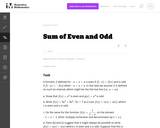Unrestricted Use
CC BY
Rating
0.0 stars

Parts (d) and (e) of this task constitute a very advanced application of the skill of making use of structure: in (d) students are being asked to use the defining property of even and odd functions to manipulate expressions involving function notation. In (e) they are asked to see the structure in the system of two equations involving functions.

Subject:
Mathematics
Secondary Mathematics
Material Type:
Activity/Lab
Provider:
Illustrative Mathematics
Provider Set:
Illustrative Mathematics
Author:
Illustrative Mathematics
05/01/2012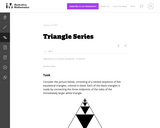Unrestricted Use
CC BY
Rating
0.0 stars

The purpose of this task is to emphasize the adjective "geometric" in the "geometric" series, namely, that the algebraic notion of a common ratio between terms corresponds to the geometric notion of a repeated similarity transformation.

Subject:
Mathematics
Secondary Mathematics
Material Type:
Activity/Lab
Provider:
Illustrative Mathematics
Provider Set:
Illustrative Mathematics
Author:
Illustrative Mathematics
05/01/2012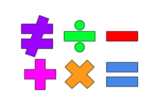Unrestricted Use
Public Domain
Rating
0.0 stars

This lesson is for a math classroom, but can be adapted to fit any grade, subject, or content.&nbsp;&nbsp;In this lesson, students will use an iPad and its features: Keynote, Pages, Garageband, Numbers, Presentation, and iMovie.&nbsp; Students will use 3 of those features/programs to create a video lesson consisting of several examples from a topic of their choice.&nbsp; This project is in place of a term final, so their chosen topic should be from their current term.Image citation: The image is one I created.&nbsp;&nbsp;

Subject:
Secondary Mathematics
Material Type:
Assessment
Author:
Tyrani Bevell